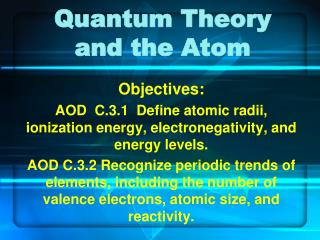# Quantum Theory and the Atom - PowerPoint PPT PresentationDownload PresentationQuantum Theory and the Atom

Download Presentation## Quantum Theory and the Atom

- - - - - - - - - - - - - - - - - - - - - - - - - - - E N D - - - - - - - - - - - - - - - - - - - - - - - - - - -
##### Presentation Transcript

1. Quantum Theory and the Atom Objectives: AOD C.3.1 Define atomic radii, ionization energy, electronegativity, and energy levels. AOD C.3.2 Recognize periodic trends of elements, including the number of valence electrons, atomic size, and reactivity.

2. Niels Bohr and Energy States • Proposed a quantum model for hydrogen to explain its discontinuous emission spectrum • Proposed that the hydrogen atom has only certain allowable energy states • Ground state: lowest allowable energy state • Excited state: when an atom gains energy

3. Niels Bohr and Energy States (cont.) • The smaller the electron’s orbit, the lower its energy state, or level. (ladder rungs) • Each energy level has a quantum number,n, applied to it. (ladder rungs) • How many electrons does hydrogen have? • In which orbit, or energy level, would you expect to find it? • This would be hydrogen’s ground state.

4. Niels Bohr and Energy States (cont.) • Bohr assigned 7 energy levels to hydrogen. • When energy is added to an atom (i.e., electric current in the lab), the electron moves to a higher-energy orbit (excited state.) • How many spectral lines did you see for hydrogen?

5. Niels Bohr and Energy States (cont.) • Spectral lines are created by energy released as an electron moves from an excited state to a lower state. • Why do we only see 4 spectral lines from H, instead of 7? • Answer: We only see the lines in the visible, ultraviolet, and infrared regions of the electromagnetic spectrum.

6. Excited Elements Lab Review • How many lines did hydrogen have? • How many lines did helium have? • How many lines did your unknown element have? • Compare to next slide.

7. Atomic Orbitals • Def: a 3-dimensional region around the nucleus • Insert Fig. 5-13, P. 132 • The more dense the cloud, the higher the probability of finding an electron there.

8. Hydrogen’s Atomic Orbitals • As you can see, the boundaries are fuzzy, so there is no defined orbital path or size. • Chemists “draw” an orbital’s surface to represent the area in which an electron spends 90% of its time. • Figure 5-13b shows the “boundaries” of the hydrogen electron’s lowest-energy orbit .

9. Hydrogen’s Atomic Orbitals (cont.) • Bohr: quantum numbers (n) • Quantum mechanical model: principal quantum numbers (n) = principal energy levels. • As n increases, the orbital becomes larger, the electron spends more time farther from the nucleus, and the atom’s energy level increases. (see Fig. 5-15a, p.133)

10. Hydrogen’s Atomic Orbitals (cont.) • Again, 7 energy levels have been detected for hydrogen, but in which level would the electron be found if the atom is in its ground state? • Principal energy levels contain energy sublevels s, p, d, and f,as follows:

11. Hydrogen’s Atomic Orbitals (cont.) • Insert table 5-2, P.134 • Relate to Electron Configuration Concert activity.

12. Hydrogen’s Atomic Orbitals (cont.) • How many sections have a Row 1? • How many boxes in each row of Section s? • How many seats in each box? • How many sections have a Row 2? • Which sections are they? • How many boxes in each row of Section p? • How many seats in each box?

13. Hydrogen’s Atomic Orbitals (cont.) • Row # (principal energy level) = n • # of sections (sublevels) with that Row # increases by 1 each time • # of boxes (orbitals) in each Row # = n2 • How many seats are in each box??? • So # of seats (electrons) in each Row # (including ALL sections) = 2n2 • Insert Table 5-2 again.

14. What Do You Know? • The lowest allowable energy state of an atom is called its ____________. • According to Bohr’s atomic model, the larger an electron’s orbit, the _______ the atom’s energy level. • What did Bohr propose happens when energy is added to a hydrogen atom? • Study Guide: p.28 (15-22, omit 17)